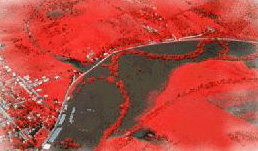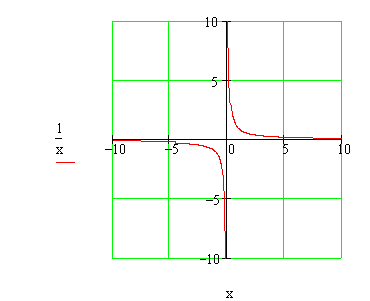Calculus on Demand at Dartmouth College Lecture 6 | Index | Lecture 8 Lecture 7## Resources

Math 3 Course Syllabus
Practice Exams

# Contents

In this lecture we discuss the concept of the limit of a function at a point, and of the limit of a function at plus infinity or minus infinity.

### Quick Question

If we define f(x) = 1/x, is there any value of x for which f(x) = 0?### Outline

Outlines for
The Legacy of Galileo, Newton, and Leibniz
Limits of Functions
Limits at Infinity

### Textbook

The Legacy of Galileo, Newton, and Leibniz
Limits of Functions
Limits at Infinity

### Quiz

The Legacy of Galileo, Newton, and Leibniz Quiz
Limits of Functions Quiz
Limits at Infinity Quiz

### Videos

With G(x) a piecewise defined, bumpy function:

Evaluate these limits:

Lecture 6 | Index | Lecture 8